﻿ Riveted Joints and Linear Buckling in the Steel Load-bearing StructurePublications are Open
Access in this journal
Article Versions
Export Article
• Normal Style
• MLA Style
• APA Style
• Chicago Style
Research Article
Open Access Peer-reviewed

### Riveted Joints and Linear Buckling in the Steel Load-bearing Structure

Pavol Lengvarský , Miroslav Pástor, Jozef Bocko
American Journal of Mechanical Engineering. 2017, 5(6), 329-333. DOI: 10.12691/ajme-5-6-20
Published online: December 15, 2017

### Abstract

The article deals with the theoretical basis of riveted joints and linear buckling. The steel roof structure is loaded with force effects that take into account weight of the roof structure, the weight of the roofing, the snow load and the weight of the air conditioning. For the loaded roof structure, a stress analysis is performed using the finite element method, from which the stress fields and the size of the axial forces in rods of the roof structure are obtained. These axial forces strained the riveted elements and can also cause the buckling of the longest and the most stressed rods. For this reason, a check is carried out on the riveted elements at the most stressed places of the structure, and a check is then carried out on the strut. It is clear from the results that all the riveted elements are suitable, and there is no loss stability of the rods.

### 1. Introduction

A rivet is a short cylindrical bar with a head integral to it. The cylindrical portion of the rivet is called shank or body and lower portion of shank is known as tail. The rivets are used to make permanent fastening between the plates such as in structural work, ship building, bridges, tanks and boiler shells. The riveted joints are widely used for joining light metals. The fastenings (i.e. joints) may be classified into the following two groups: permanent fastenings and temporary or detachable fastenings 1.

Column buckling is a curious and unique subject. It is perhaps the only area of structural mechanics in which failure is not related to the strength of the material. A column buckling analysis consists of determining the maximum load a column can support before it collapses. But for long columns, the collapse has nothing to do with material yield. It is instead governed by the column's stiffness, both material and geometric 2.

The article deals with the theoretical introduction for riveted joints and linear buckling in the elastic area. Subsequently, the finite element analysis of the roof structure is performed, from which output data are used to check the riveted elements and check the rods for loss of linear stability. Only static calculations are solved in the article, but it is important take into account, that residual stresses can also occur in steel supporting structures.

### 2. Riveted Joints

A relatively large number of parts of machines are shear stressed, these parts are joining elements (rivets, pins, welds, etc.). Depending on the type of construction of the joints and the method of loading, the joints elements can be stressed by shear load, also by tension and bending load. The normal stresses from tension and bending load are small compared to the shear stresses and therefore are not take into account in the technical calculations. When performing stress calculations, it is assumed that shear stresses across the shear plane are evenly distributed. This assumption is sometimes inconsistent with the reality, therefore it is compensated by the lower value of the allowed shear stresses. In case that transfer of forces to an element stressed by shear is mediated by a small surface (eg between the rivet and the connected part), the influence of the contact stresses is also applied. The contact stresses are often distributed unevenly trought the contact area. In computational practice, therefore, the assumption of a uniform distribution of these stresses over an area equal to the projection of the contact surface to the plane perpendicular to the load force is introduced. These deviations from the fact are taken into account when determining the values of the permissible stresses at the crushing. Deformation from the contact stresses is called crushing. For strength checks, we take into account not only the shear stress, but also to the tension and crushing resistance, depending on the nature of the element to be controlled 1, 2, 3, 4, 5, 6.

Check the weakness part on tension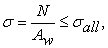(1)

where A is the area of weakened cross section, N is the axial force in this cross section and σall is the allowable stress.

The crushing resistance is defined as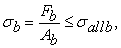(2)

where Fb is the force transmitted by the contact surface and Ab is the area of the rectangle representing the projection of the riveted on the plate section.

For riveted joints loaded axially, it is that the line of action force passes through the center of the cross section of the rivets, we assume the proportional distribution of the loading force on the individual rivets.

The strength condition for stress of rivets has form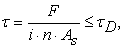(3)

where i is the number of shearing areas, n is the number of rivets and A is the shearing area.

The above relationships represent strength conditions for the stressing of the fasteners and the connected parts.

### 3. Linear Buckling

The critical force in relation to the linear buckling is called a compression force in which a beam loaded in the axial direction loses stability. In the area of elastic deformations (to the limit of proportionality), critical force and critical stress can be determined from Euler's relationship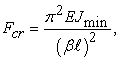(4)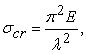(5)

where E is the Young’s modulus, Jmin is the minimal moment of inertia, λ is the bar slenderness ratio defined as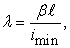(6)

where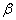is the column effective length factor depending on supports of bar ends and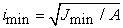is the minimum radius of gyration of the bar cross section.

The effective length factor expresses fact, how many times we have to increase length l of rod pinned on both ends, in order to have the same critical force as has the rod of length l under investigated support conditions. In Figure 1 are given some types of supports with corresponding effective length factors.

The relation (4) can be considered valid if the critical stress is within the range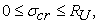where Ru is the limit of proportionality, respectively if applicable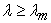(7)

while the limit magnitude of slenderness ratio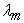is defined as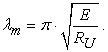(8)

The limit magnitude of slenderness ratiocreates border between buckling in elastic and elasto-plastic area. The Euler relations for critical force are applicable in elastic area, i.e. for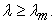For the common structural steels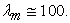If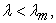i.e.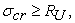we speak about buckling in elasto-plastic area, where the Euler relations for critical forces are inapplicable.

• Figure 1. Column effective length factor depending on supports of rod ends

### 4. Roof Construction

The roof truss structure consists of L profiles labeled L50x50x6, L 70x70x8, L 120x120x12 stored on support pillars. Each rod is made up of a pair of rolled L profiles (Figure 3), which are riveted to the steel joint plate usually by 3 rivets. The roof structure will be considered as a truss system where the rods are only loaded by axial forces, it is stressed by tension, respectively by compression.

• Figure 2. Roof construction

A strength analysis of the roof structure is carried out, in which the axial forces and the axial stresses in the rods are determined. The shape of the rolled L profiles is shown in Figure 4 and their basic dimensions are shown in Table 1.

• Figure 3. Rods formed by pair of L profiles
• Figure 4. Basic dimension of L profiles

The Figure 5 shows a model of the roof structure on which the boundary conditions are applied and is loaded by force effects. In the calculation model, the force F corresponds to the load applied to the area defined between the two joint plates adjacent to the vertical rods and the distance between the truss. The rod numbers are defined automatically by the finite element program and are shown in Figure 6. For the calculation, the cross sectional area of individual rods according to Table 1 is defined, the material used for the steel roof structure is 11 373. The tension strength of the material is 360 MPa and the yield strength is 235 MPa. A finite element mesh is created on the model. The used type of the element is a truss element and the element size is equal to the length of the profile.

• Figure 5. Applied boundary conditions and loads on the construction
• Figure 6. Fields of axial stresses in axis of truss

The load forces corresponding to the weight of a whole construction and the positions of the forces taking into account the skylight in the middle part of the roof structure (Figure 2). The load consists from the weight of the steel structure, the weight of the roofing and the weight of the snow or icing (F1= 32 kN + 64 kN + 160 kN = 256 kN), and from the weight of the air conditioning F2 = 2 kN acting in the joint plates II, IV and V (Figure 5). Figure 6 shows the stress fields in the individual rods for the mentioned load.

### 5. Analysis of Loaded Elements

5.1. Riveted Joints

In this section the strength analysis of the rivets used in the steel supporting structure is performed. Two types of rivets are used for riveted joints (Figure 7):

• Rivets with diameter d = 20 mm joint the L 50x50x6 and L 70x70x8 profiles to the joint plate. The shear area of the rivets with diameter d = 20mm is As = 314 mm2;

• Rivets with diameter d = 22 mm joint the L 120x120x12 profiles to the joint plate. The shear area of rivets with diameter d = 22 mm is As = 380 mm2;

In the strength analysis of the rivets, the investigators consider with the commonly used rivets material, i.e., with steel 11 343. The tensile strength of the material is Rm = 310 – 440 MPa. The value of the yield strength is equal Re = 205 MPa.

• Figure 7. The used rivets to joining the L profiles with joint plate

Each rod is joint by 3 rivets, check of rivets has been performed only for the most stressed rods. The most stressed rod is rod no. 35 (Figure 6).

To check the rivet joint, the rod no. 35 is selected with the profile L 120x120x12. The value of the axial and at the same time the shear force in the rod is Fs = 349 kN.

The shear stress in the rivet loaded by shear force is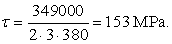(9)

We can conclude that sear stress is in elastic limit. In this case, it should be noted that beams no. 35 and no. 36 do not form separate L profiles, i.e., they are made from one rolled L profile connected from both sides with the joint plate. For this reason, the stresses in the rivets are s significantly lower.

The stress in the weakened part of the profile L 120x120x12 is equal to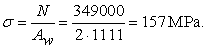(10)

Checking of rivets in the pressed rod no. 29 - profile L 50x50x6 in which the size of the axial and shear forces Fs = 10.5 kN.

The value of the shear stress in rivet loaded by shear forces is equal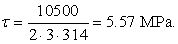(11)

We can conclude that sear stress is in elastic limit.

The tension in the weakened part of the profile L 50x50x8 is equal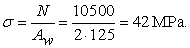(12)
5.2. Linear Buckling

For the check the rods on the linear buckling, the compression rod no. 29 (Figure 6) is selected based on the numerical calculation. The elastic modulus of the used material is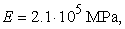, the minimal moment of inertia is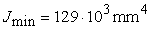and the column-effective length factor depending on supports of rod ends is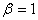(Figure 1), length of the bar is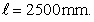Checking the conditionfor calculating the Euler's critical force.

The slenderness ratio of the rod is equal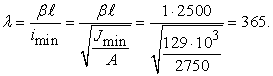(13)

The limit of the slenderness ratio of the rodis equal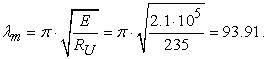(14)

The condition for calculating the Euler's critical force is fulfilled if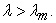(15)

Euler's critical force has value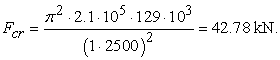(16)

The axial force in rod no. 29 has a value of 10.5 kN and the safety factor is equal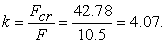(17)

The rod no. 29 will not loss of stability.

### 6. Conclusion

The introduction to the riveted joints and linear buckling theory was presented in the article. Subsequently, a stress analysis of the roof structure was performed. Where the distribution of the axial stresses and the axial forces in the rods was found. Subsequently, for the most stressed rods, the stress control was performed for the joining elements. The controlled riveted joints are suitable and will not be violation. For the rod with the highest compression stress due to its length, a linear buckling was performed where it was found that the rod is suitable. It should be noted that residual stresses play an important role in the assessment of steel load-bearing structures.

### Acknowledgements

This research was supported by a grant from the Slovak Grant Agency VEGA No. 1/0731/16, and KEGA 049TUKE-4/2017.

### References

  Meriam, J.L. and Kraige, L.G., Engineering Mechanics: Statics, Wiley, Texas, 2011. In article  Cherchuk, R.N., Method of Joints (Easy Way), Amazon Digital Services LLC, 2014, [E-book] Available: Amozon.com. In article  Bocko, J. and Delyová, I., Statics, Technical University of Košice, Košice, 2015. In article PubMed  PubMed  Jančo, R., “Numerical and exact solution of buckling load for beam on elastic foundation,” in Sborník vědeckých prací Vysoké školy báňské-Technické univerzity Ostrava, Technical University West Bohemia, 21-26. In article View Article  Lengvarský, P. and Bocko, “The Static Analysis of the Truss,” American Journal of Mechanical Engineering, 4 (7). 440-444. Dec.2016. In article View Article  Pierre, F., et al., Mechanics of materials, McGraw-Hill, New York, 2009. In article

Published with license by Science and Education Publishing, Copyright © 2017 Pavol Lengvarský, Miroslav Pástor and Jozef BockoThis work is licensed under a Creative Commons Attribution 4.0 International License. To view a copy of this license, visit http://creativecommons.org/licenses/by/4.0/